# 采用集中质量模型的大跨度斜拉桥桩–土–结构动力相互作用研究Research on Pile-Soil-Structure Dynamic Interaction of Large Span Cable-Stayed Bridge by Using Lumped Mass Model

DOI: 10.12677/HJCE.2018.74069, PDF, HTML, XML, 下载: 680  浏览: 4,411  科研立项经费支持

Abstract: In order to study the influence of pile-soil-structure dynamic interaction on seismic response about the long-span cable-stayed bridge, the seismic analysis is carried out in the background of Jiangshun Bridge of main span 700 m. The key dynamic parameters of concentrated mass model are given by M method, viscoelastic artificial boundary method and radiation damping theory. The numerical model is established by Midas/Civil, and the dynamic interaction influence law on ca-ble-stayed bridge is analyzed .Research shows that: regardless of the vertical earthquake and ho-rizontal earthquake action, the pile internal force response of the concentrated mass model is the smallest in three kinds of model, moreover, the pile top force response value is 30% smaller than that of engineering simplified model. The main reason is due to radiation damping which produces in the scattering of seismic waves propagating in infinite foundation.

1. 桩-土-结构动力相互作用研究现状

2. 集中质量模型参数的确定方法研究

2.1. 等价土体弹簧刚度的确定

Penzien通过对半空间理论分析，认为Winkler假定是成立的，因此可按照明德林(Mindlin)公式来求解土-桩相互作用的水平弹簧刚度 ${K}_{ei}$ ，但是该方法计算非常复杂  。对于实际结构，工程中可根据《公路桥涵与地基基础设计规范》 (JTG-D63 2007)，按m法计算弹性桩水平位移及作用效应。每层土弹簧的刚度K可以用下式定义：

${k}_{1}=\frac{1}{2}\left({m}_{1}{h}_{1}\right){h}_{1}{b}_{1}$ 第1层土

${k}_{2}=\frac{1}{2}\left({m}_{2}{h}_{1}+{m}_{2}{h}_{2}+{m}_{2}{h}_{1}\right){h}_{2}{b}_{1}$ 第2层土

${k}_{n}=\frac{1}{2}\left({m}_{n}\underset{1}{\overset{n-1}{\sum }}{h}_{i}+{m}_{n}{h}_{n}+{m}_{n}\underset{1}{\overset{n-1}{\sum }}{h}_{i}\right){h}_{n}{b}_{1}$ 第n层土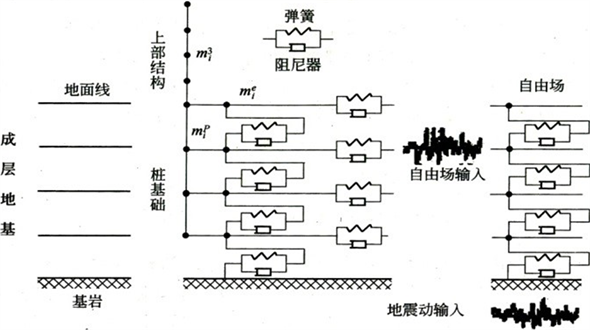Figure 1. Analysis model of the lumped mass about pile-soil-structure interaction

2.2. 自由场土层分析

${K}_{BN}={\alpha }_{N}\frac{G}{R}$${C}_{BN}=\rho {c}_{p}$ (1)

${K}_{B\tau }={\alpha }_{\tau }\frac{G}{R}$${C}_{B\tau }=\rho {c}_{s}$ (2)

2.3. 等价土体阻尼系数的确定

$\xi ={\xi }_{\mathrm{max}}\left(1-G/{G}_{\mathrm{max}}\right)$ (3)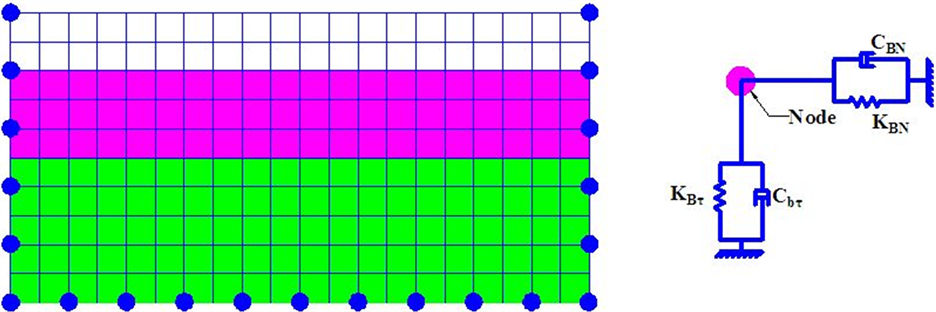Figure 2. Equivalent physical system of viscoelastic artificial boundary

$G/{G}_{\mathrm{max}}$ 与土体剪应变 $\gamma$ 之间的关系一般应根据土的工程特性试验来确定，设计时缺乏试验数据时可参考日本《道桥示方书–耐震篇》建议的方法  。该方法是在标准砂土的 $G/{G}_{\mathrm{max}}-\gamma$ 试验曲线的基础上提出的修正公式，即：

$G/{G}_{\mathrm{max}}={\left[G/{G}_{\mathrm{max}}\right]}_{1}{\sigma }_{m}^{m\left(\gamma \right)}$ (4)

${C}_{m}=2.0{\xi }_{\mathrm{max}}\left(1-\frac{G}{{G}_{\mathrm{max}}}\right)m\omega$ (5)

${C}_{r}=2A\sqrt{G\rho }\left(1+\frac{3.4}{\text{π}\left(1-\nu \right)}\right)$ (6)

$\left[{C}_{s}\right]=\left[{C}_{m}\right]+\left[{C}_{r}\right]$ (7)

2.4. 等效土体质量的确定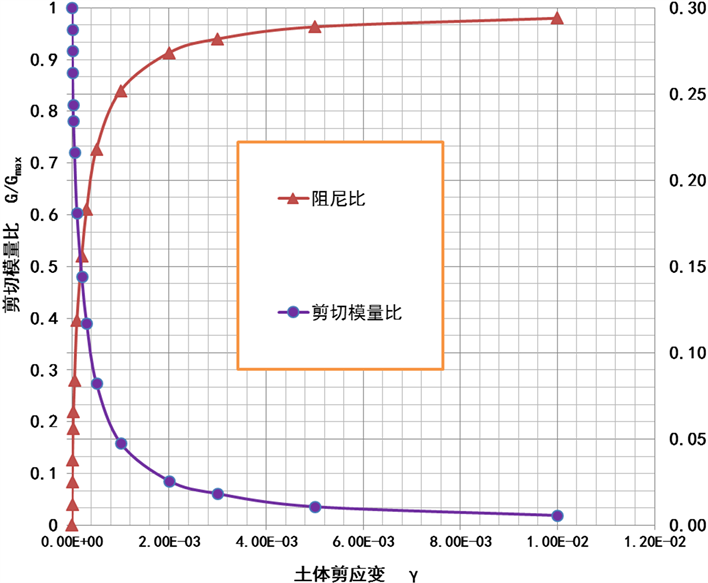Figure 3. The relationship between $G/{G}_{\mathrm{max}}$ & $\xi$ and $\gamma$

${M}_{i}^{e}={\rho }_{i}A{h}_{i}$ (8)

2.5. 层间弹簧刚度与阻尼系数的确定

${K}_{vi}=GA/{h}_{i}$ (9)

${C}_{vi}=2\left({\beta }_{i}/\omega \right){K}_{vi}$ (10)

3. 工程实例分析

1) 等价土体弹簧刚度的确定

2) 自由场土层分析和等价土体阻尼系数的确定

a) 土层自振频率 ${T}_{1}=\text{3}\text{.31}\text{\hspace{0.17em}}\text{s};\text{\hspace{0.17em}}{T}_{2}=\text{2}\text{.33}\text{\hspace{0.17em}}\text{s}$

b) 地震作用下，岩石剪应变 $\gamma$ 基本在0.001~0.002之间，覆盖层土体剪应变在0.01以上，故本桥Z3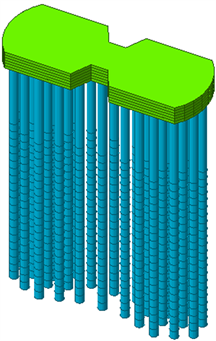(a)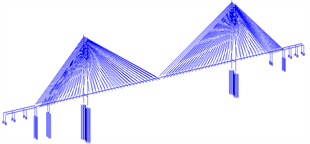(b)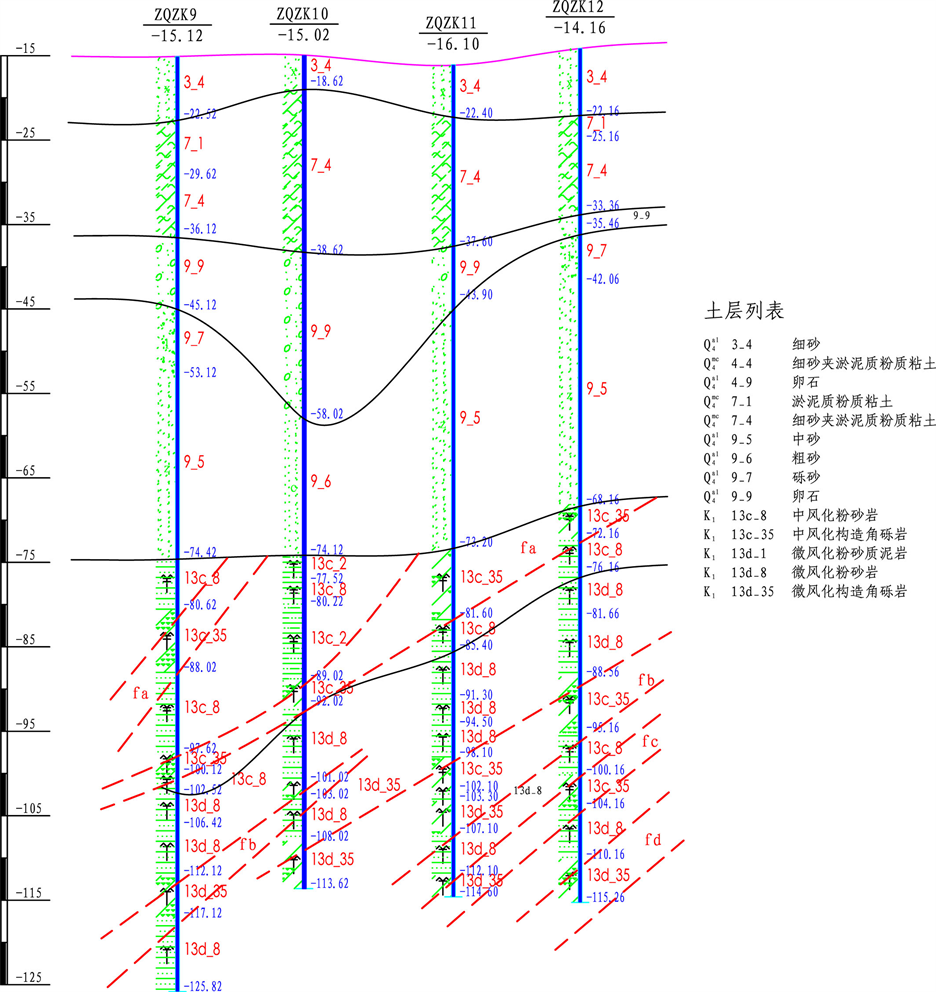(c)

Figure 4. The finite element numerical model; (a) numerical model of the whole bridge; (b) numerical model of pile cap; (c) geological drilling plan of tower

3) 等效土体质量的确定(表4)

4) 层间弹簧刚度与层间阻尼系数的确定(表5)

5) 桩–土–结构相互作用对结构地震响应的影响Table 1. The basic parameters of rock and soil mechanics of Z3 main towerTable 2. Spring stiffness coefficient of Equivalent soilTable 3. The damping coefficient of soil of Z3 main towerTable 4. Soil equivalent quality of the pileTable 5. The spring stiffness between layers and damping coefficient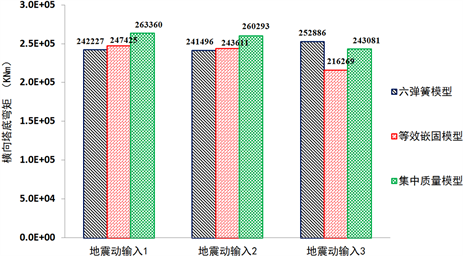(a)(b)

Figure 5. Comparative analysis of three different models with bending moment at tower bottom; (a) Longitudinal bending moment; (b) Transverse bending moment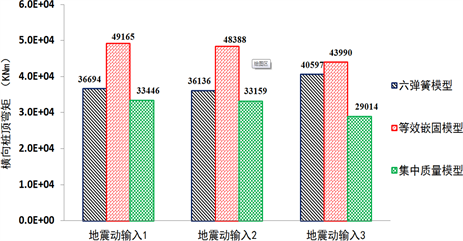(a)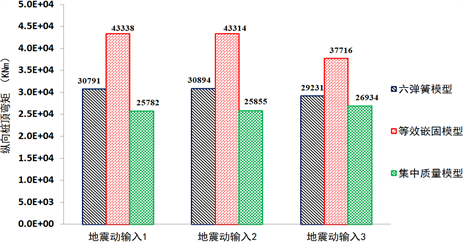(b)

Figure 6. Comparative analysis of three different models with bending moment at pile top; (a) Longitudinal bending moment; (b) Transverse bending moment

4. 结论

1) 利用m法计算土体的等效刚度，运用粘弹性人工边界法建立土体自由场模型，根据土体的粘滞与辐射阻尼理论建立土体人工阻尼，据此可确定集中质量模型的主要动力计算参数；

2) 不论是纵向地震还是横向地震作用，集中质量模型的内力响应在三种模型中是最小的，且桩顶内力响应值比简化模型最高可小30%左右；

3) 集中质量模型比简化模型计算值总体偏小的主要原因是由于地基的无限性使得结构振动的部分能量通过波的传播向无限远地基发生散射并产生辐射阻尼所致。

  林皋. 土–结构动力相互作用[J]. 世界地震工程, 1991, 7(2): 4-21.  阎韩生, 赵琦. 地震时土桩动力相互作用的Penzien模型研究[J]. 西安工业大学学报, 2008, 28(1): 76-80.  孙利民, 张晨南, 潘龙, 范立础. 桥梁土桩相互作用的集中质量模型及参数确定[J]. 同济大学学报, 2002, 30(4): 410-415.  肖晓春. 地震作用下土–桩–结构动力相互作用的数值模拟[D]: [博士学位论文]. 大连: 大连理工大学, 2003.  王满生. 考虑土–结构相互作用体系的参数识别和地展反应分析[D]: [博士学位论文]. 北京: 中国地震局地球物理研究所, 2005.  王满生, 周锡元, 胡聿贤. 土桩动力分析中接触模型的研究[J]. 岩土工程学报, 2005, 27(6): 616-620.  王有为, 王开顺. 地震作用下群桩水平刚度的计算方法及其程序设计[J]. 土木工程学报, 1984, 17(1): 41-48.  尹华伟. 土一结构动力相互作用的计算方法研究[D]: [博士学位论文]. 长沙: 湖南大学, 2005.  刘晶波, 谷音, 杜义欣. 一致粘弹性人工边界及粘弹性边界单元[J]. 岩土工程学报, 2006, 28(9): 1070-1075.  刘晶波, 王振宇, 杜修力, 杜义欣. 波动问题中的三维时域粘弹性人工边界[J]. 工程力学, 2005, 22(6): 46-51.  日本道桥协会. 道路桥示方书同解说(V耐震设计篇) [s]. 1996.  Gazetas, G., et al. (1993) Dynamic Response of Pile Groups with Different Configurations. Soil Dynamics and Earthquake Engineering, 12, 239-257. https://doi.org/10.1016/0267-7261(93)90061-U